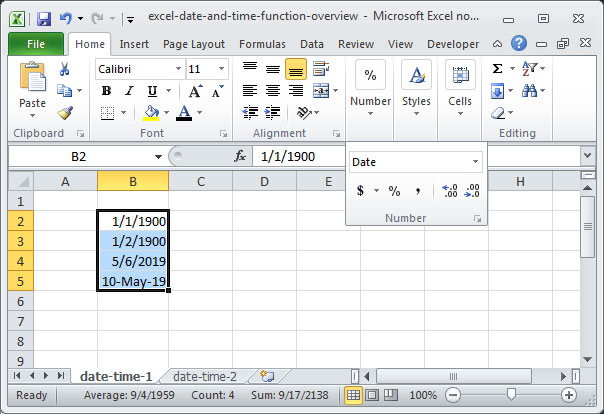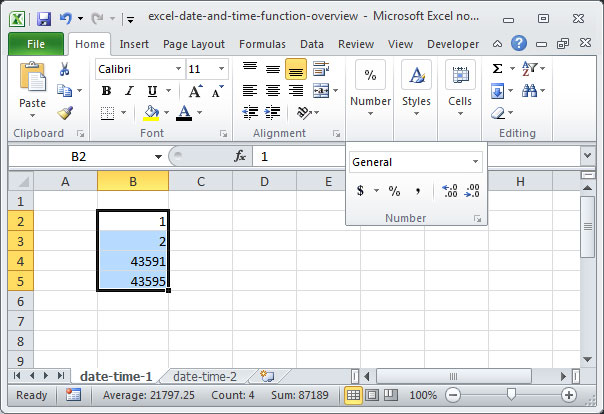# Date and time function overview

The ability to store and manipulate dates and times is one of Excel's most powerful features. Using Excel's built-in date and time functions, you can easily add days and months to particular dates; create dynamic date-based fields; and perform complex time-based calculations.

However, dates and times in Excel can be a bit difficult to wrap your head around due to the complex way in which the program stores them internally. In this tutorial, we'll take a look at the DATE and TIME functions and review how dates are stored as serial numbers in the Excel interface.

## Date storage in Excel

The most important thing to learn about dates in Excel is that they are not stored as month, day, and year combinations, but rather as encoded serial numbers that represent dates. These serial numbers are easy to interpret — they are simply the number of days that have elapsed since January 1, 1900. In other words, the date January 1, 1900 is actually stored in Excel as the number 1. The date January 2, 1900 is stored as the number 2, because it is two days away from January 1, 1900. And, the date May 6, 2019 is stored as the number 42,129, because it is 42,129 days away from January 1, 1900.

To see this phenomenon in action, enter some dates into a series of cells, like so:As you can see, Excel automatically recognizes our input as dates and formats it appropriately. But, in reality, these dates are being stored as serial numbers that represent each day's distance from January 1, 1900.

Note that we've used two different date formats above: 5/6/2019 and 10-May-2019. Excel is smart enough to recognize both of these inputs as dates and convert them to serial numbers automatically. This will even work if you enter more longform date formats into a cell — like May 10, 2019.

Let's change the number formatting on these cells from "Date" to "General" to reveal the serial numbers behind our dates:This may seem like an odd way for Excel to store dates, but the serialization process provides an important benefit: it allows us to add and subtract dates just like numbers. Let's say, for example, that we wanted to find the number of days between two dates — for example, the number of days between May 6, 2019 and May 10, 2019. Since Excel's back-end stores both dates as serial numbers, we can perform our calculation with simple subtraction:

`="5/10/2019" - "5/6/2019"Output: 4`

Likewise, we can also add a set number to a given date like so:

`="5/10/2019" + 2Output: 5/12/2019, or serial number 43,597`

## The DATE function

OK, so we know that dates are stored in Excel as serial numbers. And we know that Excel is smart enough to recognize cell inputs that look like dates and convert them to serial numbers automatically. But is there a way to generate a date's serial number formulaically?

Of course there is — the DATE function! This function takes a series of numbers representing months, days, and years as inputs, and converts the specified day into a serial number.

The syntax for DATEis as follows:

`=DATE(year, month, day)`

Fed a given year, month, and day, DATE will output the serial number equivalent to the date specified. Let's take a look at some examples of DATE in action:

`=DATE(1900, 1, 1)Output: 1/1/1990, or serial number 1`

In the above example, the formula outputs 1/1/1900, or serial number 1, because the serial number for January 1, 1900 is 1.

`=DATE(2019, 5, 6)Output: 5/6/2019, or serial number 42,129`

In the above example, the formula outputs 5/6/2019, or serial number 42,129, because the serial number for May 6, 2019 is 42,129.

## Time storage in Excel

Now that we've got a handle on dates, how should we think about storing time values in Excel? Fortunately, things here are a lot easier now that we've mastered the DATE function.

Times are also stored via serialization, but they are represented with fractional values rather than whole integers. Each day is represented by one whole serial number, and since there are 24 hours in a day, each hour is represented as 1/24 or .041666....

For the purposes of serialization, each day entered into Excel starts at 12:00am. So a date-time combination entered as 5/6/2019 8:00 would serialize as 42,129.333..., because 8:00 is eight hours past midnight, or 1/3 (.333... or 0.41666... * 8) of the day.

Note that a time value entered without a date will serialize without a leading integer — in other words, on January 1, 1900. Try entering a time, like 3:00, into a cell, and then converting the output into a date to observe this phenomenon.

If you want to use minutes and seconds in Excel, they work the same way as hours. Since a minute is 1/1,440 of a day, it is represented in Excel as 1/1,440 or 0.00069444.... And since a second is 1/86,400 of a day, it is represented in Excel as 1/86,400 or .0000115740740740....

Full time values are entered in the format, HH:MM:SS, where HH represents hours, MM represents minutes, and SS represents seconds. They can either be entered in 12-hour format or 24-hour format, so 3:00:00 PM and 15:00:00 are both valid and mean the same thing.

## The TIME function

Like the DATE function, the TIME function is used to convert a series of numbers — representing hours, minutes, and seconds — into a serialized time.

The syntax for TIME is as follows:

`=TIME(hour, minute, second)`

Provided a given hour, minute, and second, TIME will output the serial number equivalent to the time specified. Let's take a look at a few examples to see TIME in action:

`=TIME(0, 0, 0)Output: 12:00 AM, or serial number 0`

Above, the formula outputs 12:00 AM, or serial number 0, because that time is 0 hours, 0 minutes, and 0 seconds after midnight.

`=TIME(5, 23, 36)Output: 5:23:36 AM, or serial number 0.2247222...`

Above, the formula outputs 5:23:36 AM, or serial number 0.2247222..., because that time is 5 hours, 23 minutes, and 36 seconds after midnight.

Note that we can also serialize a specific date-time combination by using the DATE and TIME functions in tandem and summing the results, like so:

`=DATE(2019, 5, 6)+TIME(5, 23, 36)Output: 5/6/2019 5:23:36 AM, or serial number 42,129.2247222...`

Those are the basics of DATE and TIME used in Excel formulas! If you have questions or comments on the above, sound off in the comments section below. Otherwise, read on to learn about more handy Excel functions that handle dates and times.

## Save an hour of work a day with these 5 advanced Excel tricks

Work smarter, not harder. Sign up for our 5-day mini-course to receive must-learn lessons on getting Excel to do your work for you.

• How to create beautiful table formatting instantly...
• Why to rethink the way you do VLOOKUPs...
• Plus, we'll reveal why you shouldn't use PivotTables and what to use instead...